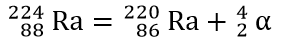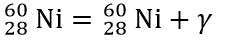# 7.1 Discrete Energy and Radioactivity

#### Discrete energy levels (n)

• Definition: discrete (specific) energy values at which the electrons of an atom may be, which correspond to "allowed" orbitals.

• Energy levels: E ≤ 0, according to the energy supplied to take an electron out of the atom (ionization).

• Ground state (n = 1): ​Normal level.

• Levels above ground state (n > 1): Electron is excited.

• n = 2 (first excited state), n = 3 (second excited state), ...​

• Ionization state (n = ∞): Energy supplied = Ionization energy.

Transition between energy levels

• Definition: electrons jumping from one level to another, emitting photon(s).

• Jumping to a higher level: An electron must receive exactly the right amount (difference between 2 energy levels) from one photon to jump to a higher energy level.

• Relaxation: Rapidly, after jumping to a higher level, the electron goes to a lower energy level  and emits a photon with energy equal to the energy difference between levels:

• Photon (γ): quantum or bundle of energy, having a specific wavelength and frequency.

• Photon energy: E = hf = hc/λ, where h = Planck's constant = 6.63 x 10^-34 Js.

Spectra

• Continuum: all wavelengths within the visible range (e.g. light bulb or Sun).

• Emission: discrete wavelengths corresponding to energy levels in which the photons are emitted. (e.g. gas exposed to strong electric field).

• Absorption: black lines are the wavelengths absorbed by the gas

• For a specific gas: Emission spectra + Absorption spectra = Continuous spectra.

• Evidence for energy levels.

Nuclear structure

Nucleons: protons and neutrons.

## Atom: nucleus + electrons

proton

neutron

electron

• Representation of a nucleus: ​• Example: Helium nucleus/alpha particle:• Number of neutrons (N) = A - Z​.

• Nuclide: "nucleus with a specific number of protons and neutron."

• Isotopes:"nuclei (plural of nucleus) with the same number of protons, but different number of neutrons"

• Conservation: charge, mass, and momentum are always conserved.

• Alpha decay (discrete energy): alpha particle (α) emission.• Beta minus decay​ (continuous energy): neutron turns into a proton, emitting an electron (called beta minus particle, β-, before the conclusion it was an electron) and an electron anti-neutrino.• Beta plus decay (continuous energy): proton turns into a neutron, emitting a positron (the anti-particle of the electron), also called the beta plus particle, β+, and a electron neutrino.• Gamma decay (discrete energy): emission of a photon (γ) of high frequency, and thus high energy, often accompanied by an alpha or a beta decay.• Penetrative power: ​α < β < γ.

• Ionization: ​γ < β < α.

• "Inverse process": Artificial transmutation: particle fired at a nucleus, leading to the formation of a new element.

Laws of radioactive decay

• Constant probability of decay.

• Rate of decay: proportional to the number of nuclei that have not yet decayed.

• Activity (A): number of decays per second, in becquerel (Bq).

• Half-life: "interval of time after which the activity/number of parent nuclei of a radioactive sample is reduced by a factor of two."

• Exponential decrease:

Radioactive decay simulation starting with 4 or 400 atoms.

• Background radiation: radioactivity activity of all other sources, e.g. cosmic rays from the Sun, which needs to be subtracted when measuring the activity of a certain radioactive sample.

Fundamental forces

Four types of interaction between particles

# 7.2 Nuclear Reactions

Patterns for stability in nuclides

• Nuclides with low proton number:  stable when the neutron-proton ratio is close to one.

• Heavier elements: the neutron-proton ratio gradually increases, due to the attractive nature of the strong force between nucleons, but repulsive nature of the electromagnetic force between protons.

Binding energy

• Mass defect (δ): the difference between the total mass of the individual nucleons making up a nucleus and the actual mass of the nucleons.

• δ = total mass of nucleons - mass of nucleus.

• If it is an atom, remember to subtract the mass of electrons.

• Einstein's theory of relativity (relationship between mass and energy): E = mc^2.

• Binding energy definition: "the minimum energy required to completely separate the constituent nucleons of one nucleus".

• Binding energy = δc^2, i.e. the mass defect transformed into energy by E = mc^2.

• Graph: average binding energy per nucleon x nucleon number.

• Average binding energy for H is zero (only one nucleon).

• Peak: Maximum average binding energy per nucleon for Fe and Ni, especially 56 - Fe, the most stable nucleus.

• The curve drops gently after the peak.

• Explanation: A given nucleon can only interact with its near neighbors. Hence, for large nuclei, all nucleons interact with roughly the same number of nucleons.

fission

fusion

Energy released in a decay

• Mass difference (∆m) must be calculated to determine whether a nuclear reaction happens.

• ∆m = total mass of reactants - total mass of products. From this, energy can be calculated, using E = mc^2.

• If ∆m > 0, the reaction releases energy.

• If ∆m < 0, the reaction needs energy to be supplied.

Nuclear fission

• Definition: heavy nuclei (nucleon number > 56) splits up into lighter nuclei.

• Induced reaction: A slow moving neutron may collide with a fissile nucleus and trigger a fission reaction.

• Chain reaction: The production of neutrons is a feature of fission reactions, which may trigger a other reactions, and thus, a chain reaction.

• Critical mass: needed to trigger chain reaction, otherwise the neutrons will escape.

• Fission reaction types: Fission reactor: slowly; Atomic fission bomb: rapidly.

• Energy of the reaction given in the form of kinetic energy of the products and as gamma radiation.

Nuclear fusion

• Definition: joining two light nuclei (nucleon number < 56) into a heavier one.

• Sun conditions: high temperatures and pressure, to overcome coulombic repulsion.

and energy..!

Deuterium

Tritium

Ethics and morals

• Nuclear energy: dangerous knowledge, since it may be used to craft atomic bombs.

• Examples: radioactivity at Hiroshima and Nagasaki.

neutron + energy + He

# 7.3 The Structure of Matter

Thomson's "plum pudding" model of the atom

• A number of electrons buried in a cloud of positive charge.

spherical cloud of positive charge

negatively charged electron

Rutherford, Geiger and Marsden experiment

• Alpha particles directed at a thin gold foil in a vacuum chamber.

• Vacuum chamber: avoid​ collisions with air particles

• Thin foil: avoid multiple deflections.

• Results: great majority of particles passed through the gold foil with little or no deviation, but, occasionally, some alpha particles were detected at large scattering angles.

• Thomson's model: only the small deflections could be understood.

• Consequences: there must be an enormous force to reflect alpha particles, which means that the positive charge is not distributed, but concentrated in a small positive nucleus.

• New model!

Anti-particles

• Definition: have the same mass as their corresponding particles, but all other properties are opposite, such as electric charge. They are denoted by a bar on the top.

• Particle annihilation: when a particle and its corresponding anti-particle collide, they annihilate, producing energy.

Elementary particles (according to the Standard model)

• Definition: particles that are, theoretically, not made out of any smaller components.

Quarks: have six different types of flavors, split into three generations of increasing mass.

• Hadron: particles built from quarks​.

• Baryon: made out of 3 quarks, e.g. proton (uud) or neutron (udd).

• Meson: made out of a quark and an anti-quark, e.g. pions.

• Quark confinement: It is not possible to observe isolated quarks. Interacting by the strong nuclear force, moving them away stores more energy in the interaction, and thus, more energy is needed to free them.

• Baryon number (B): For quarks: +1/3; For anti-quarks: -1/3.

• Hence, for baryons: +1; for mesons: 0.​

• Strangeness: indicates a surprisingly long lifetime (10^-10 s instead of 10^-15 s).​

• Conservation only in strong interactions.​

Leptons: six different types, split into three generations of increasing mass.

increasing mass

• No confinement: found out by themselves, since they interact by the weak interaction.

• Lepton number (L): For leptons and neutrinos: +1; For their antiparticles: -1.

Exchange particles: "virtual" particles, not detected during the exchange. Their mediator effect, however, is felt by the interacting particles, e.g. force between particles, transfer energy etc.

• Analogy: Invisible ball.

Feynman diagrams

• Use: represent particles interactions.

this wavy line represents the exchange of a virtual particle

The Higgs particle

It is a particle responsible, through its interactions, for the mass of the particles of the Standard Model.